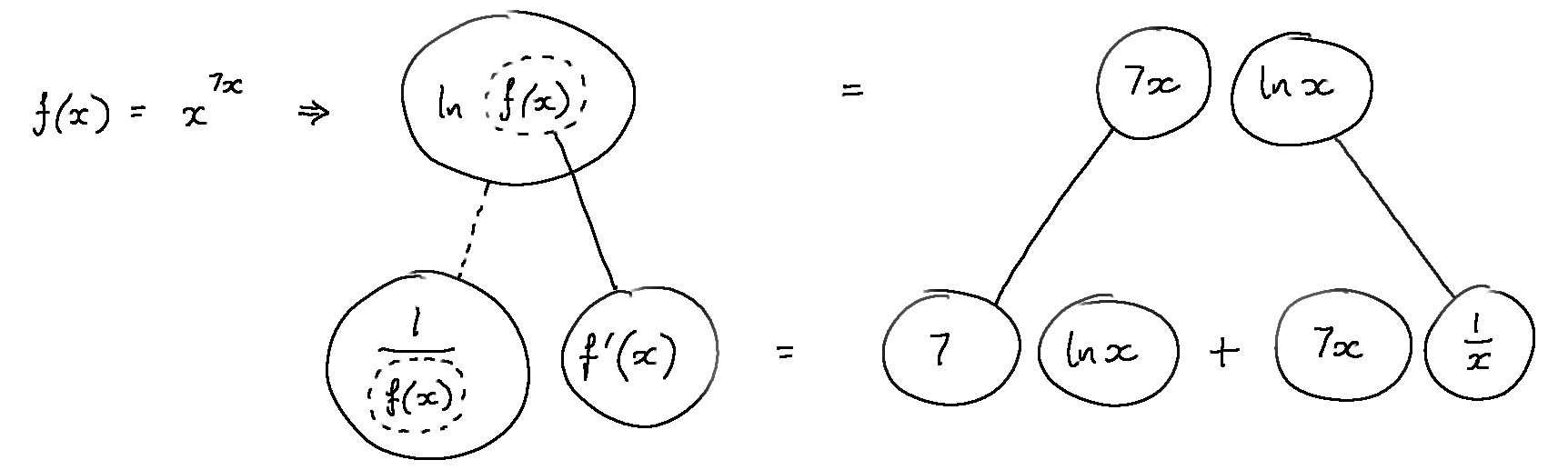# Thread: Help on log derivation

1. ## Help on log derivation

1. find f'(x) ofI gotusing the logarithmic rule and the product rule right?

2. If, find.

What do I do first? Take the ln so I get the ^x in front of the ln? Thanks

2. 1. The right direction - you've basically done...... where (key in spoiler) ...

Spoiler:... is the chain rule. Straight continuous lines differentiate downwards (integrate up) with respect to the main variable (in this case x), and the straight dashed line similarly but with respect to the dashed balloon expression (the inner function of the composite which is subject to the chain rule). And...... is the product rule, straight continuous lines still differentiating downwards with respect to x.

... but you've not finished solving the bottom row for f'(x)...

7 ln x you've multiplied by f(x) but not the other term (the one cancelling down to 7).Originally Posted by softballchick2. If, find.

What do I do first? Take the ln so I get the ^x in front of the ln? Thanks
Yes - so you could draw a similar picture?
_________________________________________

Don't integrate - balloontegrate!

Balloon Calculus; standard integrals, derivatives and methods

Balloon Calculus Drawing with LaTeX and Asymptote!

3.Originally Posted by softballchick1. find f'(x) ofI gotusing the logarithmic rule and the product rule right?

No, that's NOT right. How did you get it?

2. If, find.

What do I do first? Take the ln so I get the ^x in front of the ln? Thanks
ln(f(x))= ln(8)+ xln(sin(x))

4. whenever you are told to differentiate something like in Q1 and Q2, take the natural logarithm of both sides.

$\displaystyle y = x^{7x}$
take natural logarithm
$\displaystyle ln y = 7x . lnx$
differentiate implicitly. on RHS, use product rule
$\displaystyle \frac{y'}{y} = 7 lnx + 7x . \frac{1}{x}$
$\displaystyle y' = x^{7x}.(7lnx + 7)$

remember that when you multiply by y over to the other side, 7lnx and 7x both get multiplied

5. Hello, softballchick!

$\displaystyle \text{2. If }f(x) \:=\:8(\sin x)^x,\:\text{ find }f'(2)$

$\displaystyle \text{Let }\,y \;=\;8(\sin x)^x$

$\displaystyle \text{Take logs: }\:\ln y \:=\:\ln\left[8(\sin x)^x\right] \;=\;\ln 8 + \ln(\sin x)^x \;=\;\ln8 + x\ln(\sin x)$

$\displaystyle \text{Differentiate implicitly: }\:\dfrac{1}{y}\cdot y' \;=\;x\cdot\dfrac{\cos x}{\sin x} + \ln(\sin x)$

. . . $\displaystyle \dfrac{y'}{y} \;=\;x\cdot\cot x + \ln(\sin x)$

. . . $\displaystyle y' \;=\;y\left[x\cdot\cot x + \ln(\sin x)\right]$

.$\displaystyle f'(x) \;=\;8(\sin x)^x\left[x\cdot\cot x + \ln(\sin x)\right]$

$\displaystyle \text{Therefore: }\:f'(2) \;=\;8(\sin 2)^2\left[2\cot 2 + \ln(\sin 2)\right] \;\approx\;-6.683353787$

#### Search Tags

derivation, log